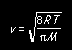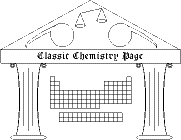## Dulong & Petit law

Early in the 19th century, two French scientists announced a law that would eventually be named after them. The law of Dulong and Petit deals with the heat capacities of elements. Later in the 19th century it would prove to be helpful in clarifying atomic weights and atomic formulas. The table below contains the data on which Dulong and Petit based their law.
 element Relative weights of the atoms (O = 1) Specific heats (cal/g°C) Bismuth 13.3 0.0288 Lead 12.95 0.0293 Gold 12.43 0.0298 Platinum 11.16 0.0314 Tin 7.35 0.0514 Silver 6.75 0.0557 Zinc 4.03 0.0927 Tellurium 4.03 0.0912 Copper 3.957 0.0949 Nickel 3.69 0.1035 Iron 3.392 0.1100 Cobalt 2.46 0.1498 Sulfur 2.011 0.1880
1) Express the data in modern units: the atomic weights in g/mol and the specific heats in J/g°C. (For atomic weights, oxygen is the key to the conversion factor: 1 DP unit = 16.00 g/mol.)

2) Is there a relationship between specific heat and atomic weight? If so, what is it? Try plotting the specific heat (call it c) vs. the atomic weight (call it M), then ln c vs. M, and then ln c vs. ln M. (Use modern units in these plots.) Is the result of any of the plots a straight line? If so, note the slope m and y-intercept b, and write the resulting equation y = mx + b in terms of c and M. Finally, solve that equation for c as a function of M: that is the relationship between specific heat and atomic weight.

3) Dulong and Petit expressed the relationship in a different but equivalent way. They noted that the product of atomic weight and specific heat was very nearly constant. Furthermore, they recognized that the product represents the atomic heat capacity (or molar heat capacity, as we would say). As Dulong and Petit put it, "The atoms of all simple bodies have exactly the same capacity for heat."
a) Multiply the specific heat by atomic weight (in modern units) for each element in the table above.
b) What are the units of the product?
c) How constant are the molar heat capacities that result in part 3a? Compute their mean and standard deviation.

### Reference

Alexis-Thérèse Petit & Pierre-Louis Dulong, "Research on some important aspects of the theory of heat," Annales de Chimie et de Physique 10, 395-413 (1819).
Copyright 2003 by Carmen Giunta. Permission is granted to reproduce for non-commercial educational purposes.Back to the Classic Calculations home pageBack to the top of the Classic Chemistry site